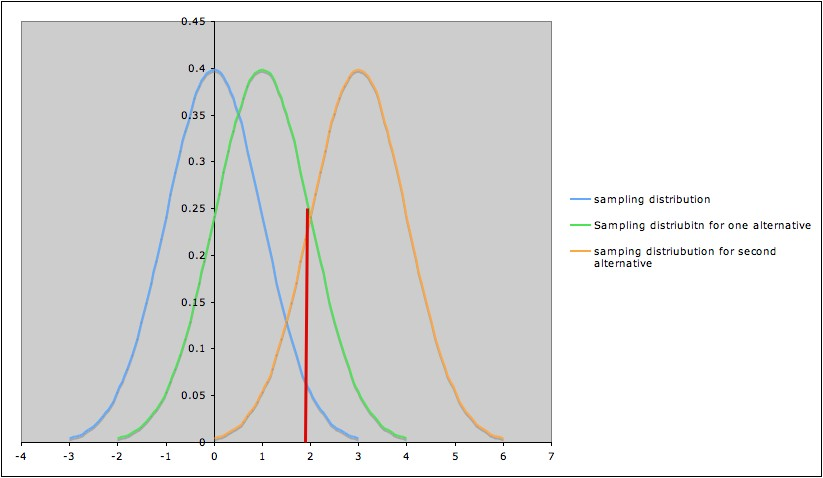COMMON MISTEAKS MISTAKES IN USING STATISTICS: Spotting and Avoiding Them

## Power of a Statistical Procedure

"... power calculations ... in general are more delicate than questions relating to Type I error."
B. Efron (2010), Large-Scale Inference: Empirical Bayes Methods for Estimation, Testing, and Prediction, Cambridge, p. 85
Overview

The power of a statistical procedure can be thought of as the probability that the procedure will detect a true difference of a specified type. As in talking about p-values and confidence levels, the reference category for "probability" is the sample.
So spelling this out in detail:

Power is the probability that a randomly chosen sample satisfying the model assumptions will detect a difference of the specified type when the procedure is applied, if the specified difference does indeed occur in the population being studied.

Note also that power is a conditional probability: the probability of detecting a difference, if indeed the difference does exist.

In many real-life situations, there are reasonable conditions that we would be interested in being able to detect, and others that would not make a practical difference.

Examples:
• If you can only measure the response to within 0.1 units, it doesn't really make sense to worry about falsely rejecting a null hypothesis for a mean when the actual value of the mean is within less than 0.1 units of the value specified in the null hypothesis.
• Some differences are of no practical importance -- for example, a medical treatment that extends life by 10 minutes is probably not worth it.
In cases such as these, neglecting power could result in one or more of the following:
• Doing much more work than necessary
• Obtaining results which are meaningless,
• Obtaining results that don't answer the question of interest.

Elaboration

For a confidence interval procedure, power can be defined as the probability1 that the procedure will produce an interval with a half-width of at least a specified amount2.

For a hypothesis test, power can be defined as the probability1 of rejecting the null hypothesis under a specified condition.
Example: For a one-sample t-test for the mean of a population, with null hypothesis H0: µ = 100, you might be interested in the probability of rejecting H0 when µ ≥ 105, or when |µ - 100| > 5, etc.

As with Type II error, we need to think of power in terms of power against a specific alternative rather than against a general alternative.

Example: If we are performing a hypothesis test for the mean of a population, with null hypothesis H0: µ = 0, and are interested in  rejecting Ho when µ > 0, we might (depending on the situation -- i.e., on what difference is of practical significance) calculate the power of the test against the specific alternative H 1: µ = 1, or against the specific alternate H3 : µ = 3, etc. The picture below shows three sampling distributions:
• The sampling distribution assuming H0 (blue; leftmost curve)
• The sampling distribution assuming H1 (green; middle curve)
• The sampling distribution assuming H3 (yellow; rightmost curve)The red line marks the cut-off corresponding to a significance level α = 0.05.
• Thus the area under the blue curve to the right of the red line is 0.05.
• The area under the green curve the to right of the red line is the probability of rejecting the null hypothesis (µ = 0) if the specific alternative H1: µ = 1 is true. In other words, this area is the power of the test against the specific alternative H1: µ = 1. We can see in the picture that in this case, this power is greater than 0.05, but noticeably less than 0.50.
• Similarly, the area under the yellow curve the to right of the red line is the power of the test against the specific alternative H3: µ = 3. Notice that it is much larger than 0.5.
This illustrates the general phenomenon that the farther an alternative is from the null hypothesis, the higher the power of the test to detect it. 3

Note: For most tests, it is possible to calculate the power against a specific alternative, at least to a reasonable approximation, if relevant information (or good approximations to them) is available.  It is not usually possible to calculate the power against a general alternative, since the general alternative is made up of infinitely many possible specific alternatives.

Power and Type II Error

Recall that the Type II Error rate
β of a test against a specific alternate hypothesis test is represented in the diagram above as the area under the sampling distribution curve for that alternate hypothesis and to the left of the cut-off line for the test. Thus

(Power of a test against a specific alternate hypothesis) + β = total area under sampling distribution curve = 1,
so
Power = 1 - β

Factors that Influence Power

In addition to the alternative or other degree of difference (e.g., width of confidence interval) desirable to detect, sample size, variance, and experimental design influence power. More

Detrimental Effects of Underpowered or Overpowered Studies

Notes:
1.  Again, the reference category for the probability is the samples.

2. This assumes a confidence interval procedure that results in a confidence interval centered at the parameter estimate. Other characterizations may be needed for other types of confidence interval procedures.

3.
The Rice Virtual Lab in Statistics' Robustness Simulation can be used to illustrate, in an interactive manner, the effect of the difference to be detected (and also of standard deviation), on power for the two-sample t-test.

Last updated August 28, 2012